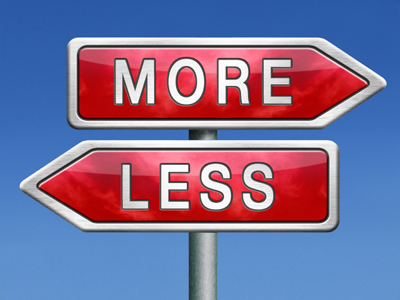If you are adding then you get more. If you are taking away then you get less.

This Math quiz is called 'Addition and Subtraction Language' and it has been written by teachers to help you if you are studying the subject at elementary school. Playing educational quizzes is a fun way to learn if you are in the 1st or 2nd grade - aged 6 to 8.

It costs only \$12.50 per month to play this quiz and over 3,500 others that help you with your school work. You can subscribe on the page at Join Us

In elementary school children are taught the language associated with the number operations of addition and subtraction, which is wide and varied. It can also be confusing - for example, to find the difference between two numbers, it's possible to count up from the lower to the higher number (effectively adding) or count down from the higher to the lower (subtracting)! It's worth knowing the key language associated with the concept of addition or subtraction. This will be especially useful when tackling word problems.

How well do you understand addition and subtraction vocabulary?

1.
Which of the following is to do with addition?
subtract
take
minus
total
A total is the sum of two or more groups
2.
What would you do to solve this:
Seven plus eight =
Take 7 away from 8
Take 8 away from 7
Subtract 7 from 8
Plus is another word for add
3.
Which of the following is to do with subtraction?
take away
totalling
in total
Taking one number away from another is subtracting
4.
Which word could be placed in the space?
16 ____ 4 gives an answer of 12.
divided by
plus
minus
16 - 4 = 12. Minus is another word for subtract
5.
Which words tell you this is an addition:
I had three balls and I bought six more. How many did I have altogether?
Three and six
More and altogether
Balls and many
Bought and have
More and altogether suggest adding up
6.
Which of the following could be used for addition or subtraction?
minus
the difference between
plus
subtract
The difference between two numbers can be found by either adding or subtracting
7.
Which of the following is to do with addition?
sharing
put together
take away
multiply
Putting two or more groups together is the same as adding them
8.
Which words could be placed in the space?
8 ____ 11 gives an answer of 3.
multiplied by
divided by
subtracted from
Sometimes the number to be subtracted can come first in the number sentence
9.
Which word could be placed in the space?
12 ____ 8 gives an answer of 20.
subtract
plus
minus
multiplied by
Plus is another word for add
10.
Which of the following is to do with subtraction?
less than